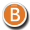• 3D Figure Assets

By:  WiwimaX ()

11.99 USD• 3D Figure Assets

By:  WiwimaX ()

11.95 USD• 3D Figure Assets

By:  WiwimaX ()

13.95 USD• 3D Figure Assets

By:  WiwimaX ()

11.95 USD• 3D Figure Assets

By:  WiwimaX ()

10.95 USD• 3D Figure Assets

By:  WiwimaX ()

11.95 USD• 3D Figure Assets

By:  WiwimaX ()

10.95 USD• 3D Models

By:  WiwimaX ()

14.95 USD• 3D Figure Assets

By:  WiwimaX ()

9.85 USD• 3D Figure Assets 3D Models

By:  WiwimaX ()

12.95 USD• 3D Figure Assets

By:  WiwimaX ()

11.95 USD• 3D Figure Assets

By:  WiwimaX ()

12.85 USD• 3D Figure Assets

By:  WiwimaX ()

12.99 USD• 3D Figure Assets

By:  WiwimaX ()

9.85 USD• 3D Figure Assets

By:  WiwimaX ()

11.95 USD• 3D Figure Assets

By:  WiwimaX ()

10.50 USD• 3D Figure Assets 3D Models

By:  WiwimaX ()

13.95 USD• 3D Figure Assets

By:  WiwimaX ()

12.85 USD• 3D Figure Assets

By:  WiwimaX ()

10.99 USD• 3D Figure Assets

By:  WiwimaX ()

9.85 USD• 3D Figure Assets

By:  WiwimaX ()

9.85 USD• 3D Figure Assets

By:  WiwimaX ()

11.45 USD• 3D Figure Assets

By:  WiwimaX ()

13.00 USD• 3D Figure Assets

By:  WiwimaX ()

10.95 USD• 3D Figure Assets

By:  WiwimaX ()

10.95 USD• 3D Figure Assets

By:  WiwimaX ()

10.50 USD• 3D Figure Assets

By:  WiwimaX ()

12.95 USD• 3D Figure Assets

By:  WiwimaX ()

11.95 USD• 3D Figure Assets

By:  WiwimaX ()

8.95 USD• 3D Figure Assets

By:  WiwimaX ()

12.00 USD• 3D Figure Assets

By:  WiwimaX ()

13.00 USD